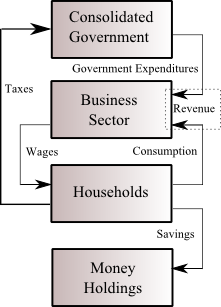## Wednesday, October 26, 2016

### Building A SFC Model In Python

This article demonstrates the first basic application of my Stock-Flow Consistent (SFC) models package in Python: sfc_models. The code is under construction, and needs to be extended in many directions. However, with the core functionality built, adding new analytic capabilities should be straightforward. In this article, I explain how to implement the simplest SFC model -- Model SIM ("SIMplest") -- from Chapter 3 of Godley and Lavoie's Monetary Economics. The key advantage of my library is that the user just specifies the high level description of the sectors of the economy, and the package generates the underlying equations.

## Model SIMFlows in Model SIM.
The model SIM is the simplest of the models in Godley and Lavoie. I needed to find external validation of the equations generated by my package, and so this was the first real test. (I have extensive unit tests of the code of the individual sectors, but we need to validate that the framework stitches the sectors together in a way that generates equations that can be solved numerically.)

The model consists of three sectors. The Household and Business sectors are tied together by the labour and goods (final output) markets, while government activity just consists of an externally set ("exogenous") demand for goods.

I have already used this model as an example multiple times, so I will not repeat the background information.If you are unfamiliar with this model, please see:

## sfc_models Package Installation

Installation instructions are found here.  (If you are familiar with Python, pip install sfc_models works.)

## Model Creation

The code to set up model SIM is as follows:

The package generates a rather impressive set of equations for such a simple model.

GOV_F = GOV_LAG_F +GOV_T -GOV_DEM_GOOD
GOV_FISC_BAL = GOV_T -GOV_DEM_GOOD
GOV_LAG_F = GOV_F (k -1 )
GOV_T = TF_T          .
HH_AfterTax = HH_PreTax -HH_T
HH_AlphaFin = 0.4000
HH_AlphaIncome = 0.6000
HH_DEM_GOOD = HH_AlphaIncome *HH_AfterTax +HH_AlphaFin *HH_LAG_F
HH_F = HH_LAG_F -HH_T +HH_SUP_LAB -HH_DEM_GOOD
HH_LAG_F = HH_F (k -1 )
HH_PreTax = HH_SUP_LAB
HH_SUP_LAB = BUS_DEM_LAB
HH_T = TF_TaxRate *HH_PreTax
BUS_DEM_LAB = GOOD_SUP_GOOD
BUS_F = BUS_LAG_F -BUS_DEM_LAB +BUS_SUP_GOOD
BUS_LAG_F = BUS_F (k -1 )
BUS_SUP_GOOD = GOV_DEM_GOOD +HH_DEM_GOOD
TF_T = TF_TaxRate *HH_PreTax
TF_TaxRate = 0.2000
LAB_DEM_LAB = BUS_DEM_LAB
LAB_SUP_LAB = LAB_DEM_LAB
GOOD_DEM_GOOD = GOV_DEM_GOOD +HH_DEM_GOOD
GOOD_SUP_GOOD = GOOD_DEM_GOOD
# Exogenous variable
GOV_DEM_GOOD =  [20. ,]*105  # (=20. for time 0 -> 104)

The beauty of the sfc_models package is that the algorithm generates the equations, and not the user. This greatly reduces the tedium and risk of error when generating a large SFC model. For example, a multi-country SFC model will have multiple goods and sectors, which creates an extremely large number of variables that look similar, but have to be carefully tracked.

## Equation Solution

The chart above is generated by running following code (which requires the matlibplot package):

```
import out_SIM_Machine_Model
from sfc_models.examples.Quick2DPlot import Quick2DPlot```
```
```
```obj = out_SIM_Machine_Model.SFCModel()
obj.main()
obj.WriteCSV('out_SIM_Machine_Model.csv')
Quick2DPlot(obj.t, obj.GOOD_SUP_GOOD, 'Goods supplied (national production Y)')```

The model solution is done within another file ("out_SIM_Machine_Model.py") which was machine-generated. Although this is somewhat eccentric, it was the safest (and most efficient) way of allowing for fairly general equation forms.

The module sfc_models.examples.scripts.build_SIM_model.py does all the steps involved in building the model.

## Next Steps

In order to be more interesting, the sfc_models package will need to add support for more complicated interactions between sectors. I want to finish off my next book ("Abolish Money!"), and will then turn more attention to that task.

(c) Brian Romanchuk 2016